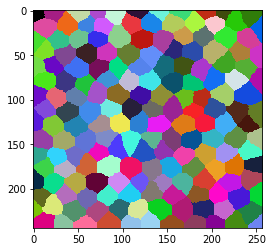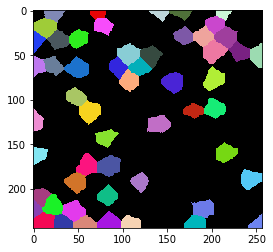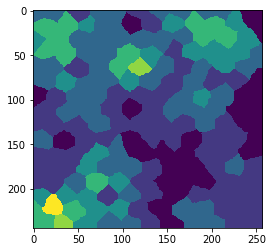# Count proximal labels in an other label image#

import numpy as np
import pyclesperanto_prototype as cle

cle.get_device()

<gfx90c on Platform: AMD Accelerated Parallel Processing (2 refs)>


Our starting point is a label image and another label image, where some of the labels in the first image are selected from.

label_image = cle.artificial_tissue_2d()
cle.imshow(label_image, labels=True)random_vector = np.random.random((1, int(label_image.max() + 1)))
sparse_labels = cle.exclude_labels_with_values_out_of_range(random_vector, label_image, minimum_value_range=0, maximum_value_range=0.3)
cle.imshow(sparse_labels, labels=True)We now count for every label in label_image, how many labels are proximal to it in the sparse_labels image. For measuring the distance, we use the centroid distance.

count_map = cle.proximal_other_labels_count_map(label_image, sparse_labels, maximum_distance=25)
cle.imshow(count_map)